﻿ 基于灰色关联法的卫星在轨关键约束影响分析

# 基于灰色关联法的卫星在轨关键约束影响分析 Analysis of Key Constraints of Satellite Orbits Based on Grey Correlation Method

Abstract: In recent years, agile optical remote sensing satellites have become the development direction of a new generation of remote sensing satellites. Agile satellites have a large number of operating parameters and performance index parameters when they are in-orbit observation. There is a certain implicit relationship between them. In this paper, the satellite in-orbit application strategy software is used to simulate the fine observation scenes of key targets, and a series of satellite on-orbit working parameters and performance index parameters are obtained. In this paper, the integration time, ground sampling distance (GSD), imaging width, imaging strip length, imaging strip number and five performance indicators are selected as the analysis objects, and the gray correlation method is used to correlate the performance index with the on-orbit parameters. The mining of rules, mining the key factors affecting various performance indicators, and analyzing the mining results, provides a basis for reducing or eliminating the constraints on the imaging performance indicators, simplifying the complexity of the simulation system, and improving the ease of use and ease of use of agile satellites laid the foundation.

1. 引言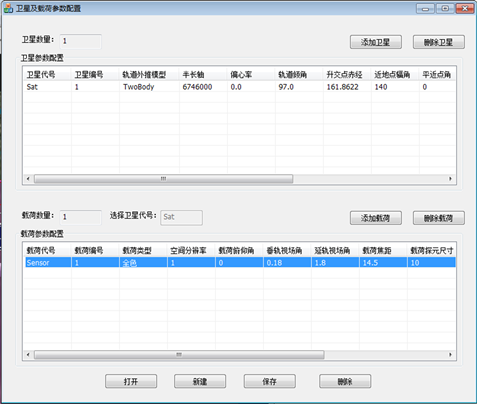(a)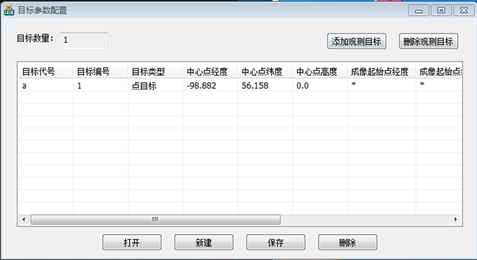(b)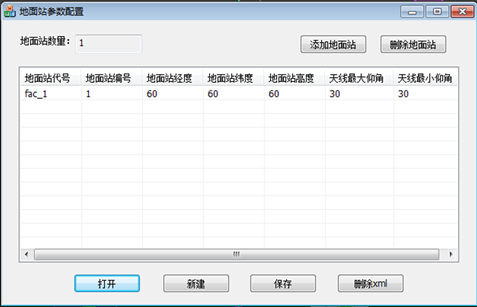(c)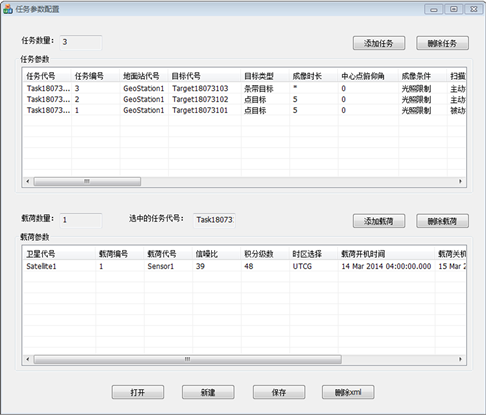(d)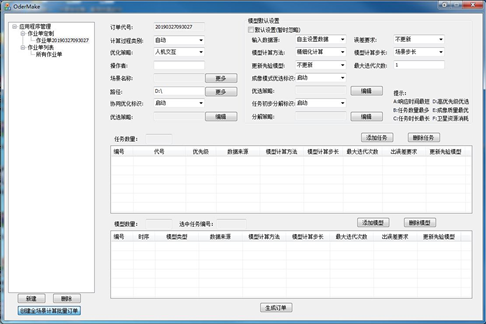(e)

Figure 1. Satellite on-orbit application strategy software simulation interface. (a) Satellite parameter setting interface; (b) Target configuration interface; (c) Ground station parameter configuration interface; (d) Task parameter configuration interface; (e) Order configuration interface

2. 重点目标精细观测参数选择

3. 灰色关联分析法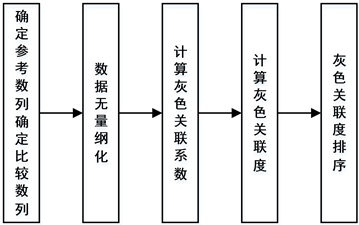Figure 2. Grey correlation algorithm flow

1) 确定系统的参考数列和比较数列：参考数列是反映系统行为特征的数列，比较数列是影响系统行为的因素组成的数列。在本文中，将卫星在轨成像效能指标作为参考数列，各在轨约束参数作为比较数列。

2) 对参考数列和比较数列进行无量纲化处理：由于系统中各数据所表示的物理意义不同，导致数据的量纲也不相同，不便于进行比较，或在比较时不易得到正确的结论。因此在进行灰色关联度计算分析时，都要事先进行数据的无量纲化处理。

3) 求参考数列与比较数列的灰色关联系数 $\xi \left({X}_{i}\right)$ ：所谓关联程度，实质上是曲线间几何形状的差别程度。因此曲线间差值大小，可作为关联程度的衡量尺度。对于一个参考数列 ${X}_{0}$ 有若干个比较数列 ${X}_{1},{X}_{2},\cdots ,{X}_{n}$ ，各比较数列与参考数列在各个时刻(即曲线中的各点)的关联系数 $\xi \left({X}_{i}\right)$ 可由公式(1)算出：

${\xi }_{oi}=\frac{\Delta \left(\mathrm{min}\right)+\rho \Delta \left(\mathrm{max}\right)}{{\Delta }_{oi}\left(k\right)+\rho \Delta \left(\mathrm{max}\right)}$ (1)

4) 灰色关联度的计算：因为关联系数是参考数列与参比较数列在各个时刻(即曲线中的各点)的关联程度值，所以它的数值不止有一个，而信息过于分散不利于对数据进行整体性的比较。因此有必要将各个时刻(即曲线中的各点)的灰色关联系数集成为一个值，即取其平均值，作为比较数列与参考数列间关联程度的数量表示，即灰色关联度 ，如公式(2)：

${r}_{i}=\frac{1}{N}{\sum }_{k=1}^{N}{\xi }_{i}\left(k\right)$ (2)

${r}_{i}$ 是比较数列 ${x}_{i}$ 对参考数列 ${x}_{0}$ 的灰关联度，也被称为序列关联度、平均关联度、线关联度。 ${r}_{i}$ 值越接近1，说明相关性越好，反之，相关性越差。

5) 关联度排序：因素间的关联程度，主要是用关联度的大小次序描述，而不仅是关联度的大小。将m个子序列对同一母序列的关联度按大小顺序排列起来，便组成了关联序，记为{x}，它反映了对于母序列来说各子序列的“优劣”关系。若 ${r}_{oi}>{r}_{oj}$ ，则称 $\left\{{x}_{i}\right\}$ 对于同一母序列 $\left\{{x}_{o}\right\}$ 优于  ，记为 ${r}_{oi}$ 表示第i个子序列对母数列特征值。由此可得到各效能指标数列的关键约束排序。

4. 卫星在轨关键约束影响分析结果

Table 1. Gray correlation table of various performance indicators and on-orbit parameters

1) 影响积分时间的关键影响约束为：摄影点经纬度、星下点经纬度、轨道平近点角、真近点角。

2) 影响GSD的关键影响约束为：卫星偏航角速度、扫描地速、斜距。

3) 影响成像幅宽的关键影响约束为：斜距、扫描地速、卫星偏航角速度、太阳帆板转角。

4) 影响成像条带长度的关键影响约束为：真近点角、平近点角、星下点纬度、摄影点纬度；影响成像条带数的关键影响约束为：真近点角、平近点角、摄影点纬度、星下点纬度。

5. 总结与展望

 胡堃, 陈刚, 孙韬, 等. 一种大倾角敏捷成像卫星影像的直接定位方法[J]. 测绘科学, 2015, 40(2): 59-65.

 段岑薇. 卫星动态成像对平台稳定度的需求分析[J]. 信息通信, 2018(8): 27-28.

 刘锋, 李琳, 孟新. 遥感卫星系统能力指标体系构建模式研究[J]. 航天返回与遥感, 2017(6): 40-45.

 张新伟, 戴君, 刘付强. 敏捷遥感卫星工作模式研究[J]. 航天器工程, 2011, 20(4): 32-38.

 佚名. 航天光学遥感技术[M]. 北京: 中国科学技术出版社, 2011.

 杨秀策, 王建荣, 周瑜. 卫星侧摆摄影对影像地面采样间隔的影响[J]. 测绘科学与工程, 2014(5): 25-29.

 Gao, J., Huang, R., Nie, K., et al. (2017) Simulation and Optimization of Optical Performance of Time-Delay-Integration Complementary Metal-Oxide-Semiconductor Image Sensor Based on Hybrid Charge-Digital Accumulation Architecture. Optical Engineering, 56, Article ID: 083108.

 胡芬, 金淑英. 高分辨率光学遥感卫星宽幅成像技术发展浅析[J]. 地理信息世界, 2017(5): 53-58.

 Barkhordari, M. and Niamanesh, M. (2018) Kavosh: An Effective Map-Reduce-Based Association Rule Mining Method. Journal of Big Data, 5, 25.
https://doi.org/10.1186/s40537-018-0129-4

 邓景毅. 事务间数值型关联规则的数据挖掘[D]: [硕士学位论文]. 广州: 暨南大学, 2003.

 李云燕. 仿真数据相关性分析方法研究[D]: [硕士学位论文]. 哈尔滨: 哈尔滨工业大学, 2011.

 胡玉伟, 马萍, 杨明, 等. 基于改进灰色关联分析的仿真数据综合一致性检验方法[J]. 北京理工大学学报, 2013, 33(7): 711-715.

 佚名. 异步不等速率航迹速率比对区间灰数关联算法的性能影响仿真分析[J]. 计算机与数字工程, 2018, 46(11): 2217-2220.

 李守林, 赵瑞, 陈丽华. 基于灰色关联分析和TOPSIS的物流企业创新绩效评价[J]. 工业技术经济, 2018(4): 12-21.

 Xie, C., Bai, J., Wen, Z., et al. (2018) Lightning Risk Assessment of Transmission Lines Based on D-S Theory of Evidence and Entropy-Weighted Grey Correlation Analysis. Energy Internet & Energy System Integration, Beijing, 26-28 November 2017, 1-6.

Top